Next: Problems Up: Equivalent Circuits Previous: Thevenin's and Norton's Theorems

## Determination of Thevenin and Norton Circuit Elements

One approach to determine the equivalent circuits is:

1. Thevenin - calculate the open-circuit voltage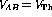.
2. Norton - calculate the short-circuit current between A and B;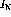.
3.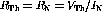.

An alternative to step 2 is to short all voltage sources, open all current sources, and calculate the equivalent resistance remaining between A and B. We will use the latter approach whenever manageable. To see if you understand equivalent circuits so far, convince yourself that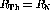.

Solution: From Thevenin's theoremAccording to Notron's theorem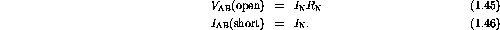Therefore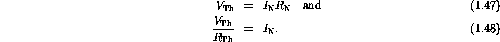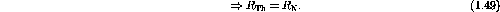Lets now return to our Wheatstone bridge example shown in figure  1.6. We will calculate the current through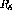by replacing the rest of the circuit by its Thevenin equivalent.

•is removed and the open terminals are labeled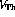. The polarity assigned is arbitrary as will be verified in the calculations.
• The evaluation ofis performed using Kirchoff's laws: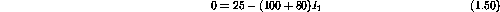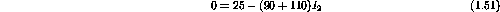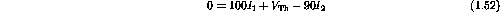The result is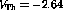V. The minus sign means only that the arbitrary choice of polarity was incorrect.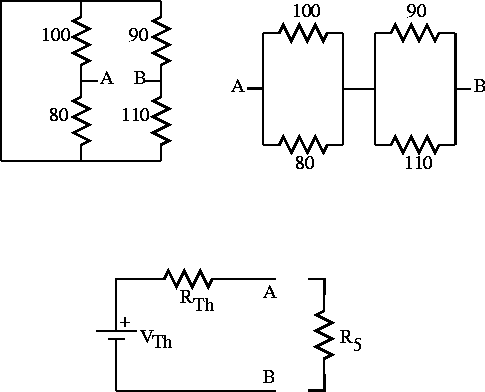Figure 1.9:   Thevenin's theorem applied to the Wheatstone bridge circuit.

• The voltage source is shorted out and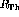is calculated (figure 1.9):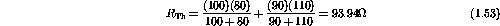Note that when the source is shorted out, the resistors that were in series (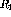and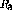;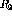and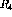) become parallel combinations.

• The network is assembled in series as shown in figure 1.9 and the current throughis calculated.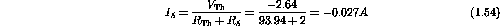Note that the numerical value of the current is the same as that in the preceding calculations, but the sign is opposite. This is simply due to the incorrect choice of polarity offor this calculation. In fact, the current flow is in the same direction in both examples, as would be expected.

Example: Find the Thevenin equivalent componentsandfor the circuit in figure 1.10.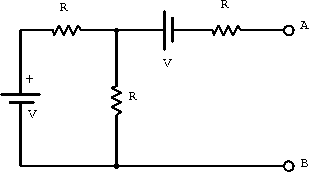Figure 1.10:  Example circuit for analysis using a Thevenin equivalent circuit.

Shorting the V's to find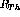gives two resistors in parallel, which are in series with a third resistor: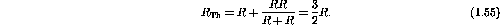The open circuit voltage gives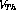. For the open circuit no current flows from the node joining the two resistors to A. A is thus at -V relative to this node. Around the interior loop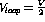(cf. voltage divider).

Therefore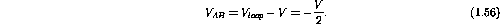Next: Problems Up: Equivalent Circuits Previous: Thevenin's and Norton's Theorems

Doug Gingrich
Tue Jul 13 16:55:15 EDT 1999